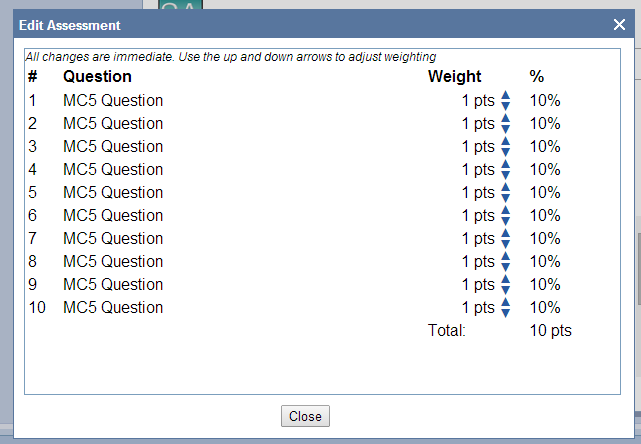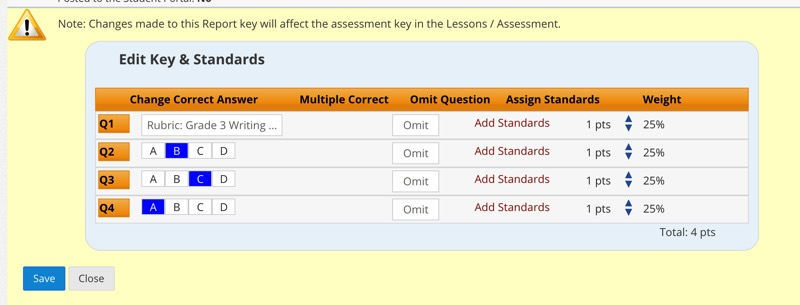# Question Weighting and Rubric Calculation

Follow
This article discusses how to weight questions and how weighting translates when grading. This topic can become confusing when grading rubrics, which use points and weighting internally and allow giving partial credit for a question. Therefore, at the bottom of this article are sections on how percents and points correlate and how rubrics and question weighting correlate.

How to Edit Question Weights

Option 1 - Before the assessment has been shared or graded:

Go to Lessons/Assessments > My Lessons > Actions > Edit, click "Edit Questions Weighting," adjust the weights using the small arrows on the right, and click Close.Option 2 - After the assessment has been graded:
Go to Reports > Sessions > Actions > Edit Key & Standards, adjust the weights using the small arrows on the right, and click Save.How Points & Percentages Work Together
Points can total any number. Points-based reports (like "Item Analysis - Points") will reflect scores in points.

Percent, in effect, represents the weight each question will have in the grade. As you adjust a question's weight, all other questions' percentages will change to add up to 100%.

TIP: It may be easier, as you adjust weights, to refer to points, because points "stay where you set them," whereas percents will constantly recalculate with each click on a weight adjustment arrow.

Some examples:
If you have 5 questions on a test and leave them at their default of 1 point, they will each be worth 20% of the grade, and missing 1 question would earn a student a grade of 80.

If those 5 questions are worth 2 points, they will each still be worth 20% of the final grade since their weight is equal, and missing one question would still result in a grade of 80.

If I set the first four questions to 1 point each, and the last question to 6 points (for a nice round total of 10 points), the first four will be worth 10% of the grade each, and the last question would be worth 60%. If I miss the first question only, I will get a grade of 90. If I miss the last question only, I will get a grade of 40.

TIP: How to set a group of questions to a combined weight:

If you have a multiple-choice section that you want to be worth 70% of the grade and there are 35 multiple choice questions in the section, dividing 70 by 35 to find the point value you should set each question to 2 points.

If you have a short answer section that you want to be worth 30% of the grade and there are 5 short-answer questions in the section, divide 30 by 5 to find the point value you should use each question to 6 points.

NOTE: Points must be whole numbers, so you may need to convert decimals to whole numbers by multiplying times ten (along with the points for every other question on the assessment to preserve relative weights).

The Relationship Between Weighting and Rubrics
It's unfortunate that both rubrics and question weighting commonly use the term "points," because points within a rubric and points in terms of question weighting affect grades in different ways.

When you first create a rubric, it is given the same weight as all other questions on the assessment. (To adjust the weight, see above.) When you grade a rubric, the criteria add up to a total number of points within the rubric. This has nothing to do with the weight of the question in the assessment - it represents what granularity of partial credit can be given for the question. If within the rubric a total of 10 possible points as available and they receive 5 points on it, the student will receive half the credit for the question; and in that case, if the question is worth 20 points in the assessment, they will receive 10 points for the question - half the credit.